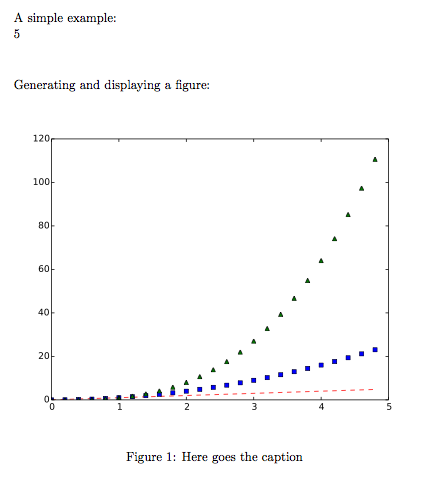## 07.12.13

### Running Python code in a LaTeX document

I am currently working on a LaTeX document in which the content is still in a very fluid phase and  the figures change often. I usually have a Python script that prepares the figures and saves them as PDFs. To iterate faster, I wanted to insert Python code directly into LaTeX, executing the code when the document is compiled.

Googling around I found that I was not the first one to want this. There is this LaTeX package with this github repo. The package provides a new environment (python) within which you can write arbitrary Python code. The code is executed when the document is compiled and the output is inserted into the document.

I try it and it worked like a charm. It was even printing the traceback in red when there was an error in the script. You can do things like this:

\documentclass[a4paper]{book}

\usepackage[pdftex]{graphicx}
\usepackage{python}

\begin{document}

A simple example:

\begin{python}
print(2+3)
\end{python}

\vspace{1cm}
Generating and displaying a figure:

\begin{python}
import numpy as np
import matplotlib.pyplot as plt

# evenly sampled time at 200ms intervals
t = np.arange(0., 5., 0.2)

# red dashes, blue squares and green triangles
plt.plot(t, t, 'r--', t, t**2, 'bs', t, t**3, 'g^')
plt.savefig('fig1.pdf', format='pdf')
print(r"""
\begin{figure}[htbp]
\centering
\includegraphics[width=12cm]{fig1.pdf}
\caption{Here goes the caption}
\label{fig:comparison}
\end{figure}
""")

\end{python}

\end{document}

and the output is:But as the document grew, the LaTeX compilation time increased dramatically. The problem: Python scripts are executed on each compilation even if they have not changed.

I have forked the repo and hacked the code. In the new version, python scripts are only executed if needed. Using a helper python script (runpy.py) to avoid encoding differences between terminals, UTF-8 is fully supported. Additionally, you can select the python binary to use and choose to save auxiliary files in a subfolder to avoid poluting the main folder. These options are explained in the README. Check the code is in this github repo.

I am not really a LaTeX programmer and therefore I am sure that there a lot of things to be improved. Feel free to send patches, suggestions and comments.

## 12.11.13

### Скрипты — diff для бинарных офисных файлов

Мне недавно захотелось странного - делать diff для файлов odt и pdf.

Скрипт вытаскивает текст из двух файлов (doc,odt,docx,pdf,xls,xlsx,ods,rtf) и сравнивает эти тексты в моей любимой программе meld.

Я применяю этот скрипт для сравнения файлов в Eclipse. Для этого надо поставить плагин (http://sourceforge.net/projects/externaldiff/). В SVN плагине Subversive уже есть функция External diff. А затем в качестве внешней команды сравнения настроить этот скрипт.

Установка зависимостей (команда для Ubuntu):
sudo apt-get install odt2txt python-pdfminer catdoc antiword unoconv

  1 2 3 4 5 6 7 8 9 10 11 12 13 14 15 16 17 18 19 20 21 22 23 24 25 26 27 28 29 30 31 32 33 34 35 36 37 38 39 40 41 42 43 44 45 46 47 48 49 50 51 52 53 54 55 56 57 #!/bin/bash DIFF_TOOL=/usr/bin/meld #DIFF_TOOL="diff -y -w" TEMP_DIR=$(mktemp -d) if [[ -z "$1" || -z "$2" || "$1" == "-h" || "$1" == "--help" ]]; then echo "Usage:$0 FILE1 FILE2" exit 0 fi function get_conv { filename=$(basename "$1") extension="${filename##*.}" extension="${extension,,}" if [[ "$extension" == "pdf" ]]; then echo "pdf2txt" elif [[ "$extension" == "doc" ]]; then echo "antiword" # echo "catdoc" elif [[ "$extension" == "rtf" ]]; then echo "catdoc" elif [[ "$extension" == "odt" ]]; then echo "odt2txt" elif [[ "$extension" == "docx" ]]; then echo "unoconv -f text --stdout" elif [[ "$extension" == "ods" || "$extension" == "xls" || "$extension" == "xlsx" ]]; then echo "unoconv -f csv --stdout" else echo "Error! Unsupported file type" exit 1 fi } filename1=$(basename "$1").txt filename2=$(basename "$2").txt command=$(get_conv "$1") $command "$1" > "$TEMP_DIR/$filename1" RESULT=$? if [$RESULT -ne 0 ]; then echo "Error!" exit 1 fi command=$(get_conv "$2") $command "$2" > "$TEMP_DIR/$filename2" RESULT=$? if [$RESULT -ne 0 ]; then echo "Error!" exit 1 fi $DIFF_TOOL "$TEMP_DIR/$filename1" "$TEMP_DIR/$filename2" rm "$TEMP_DIR/$filename1" rm "$TEMP_DIR/$filename2" rmdir "$TEMP_DIR"

## 10.11.13

### Динамические виртуальные хосты (dynamic vhost) в nginx

У apache есть такая полезная вещь как dynamic vhost - динамические виртуальных хосты. Собственно, оно нужно для того, чтобы каждый раз при добавлении домена, не было нужды редактировать файл конфигурации. Достаточно один раз настроить, добавить\обновить DNS запись и добавить директорию на сервер.
Так вот до недавнего времени, мне не было нужды в динамических виртуальных хостах, но не так давно понадобилось сделать и я, признаться, немного был поражён тому, насколько это просто, лаконично и главное гибко можно настроить в nginx.
Не буду томить, всё действительно очень просто:
server {
server_name $host; root /var/www/$host;
...
}

По большему счёту это всё, но на практике хорошо бы проверять наличие директории, и если такой нет - показывать 404 ошибку:

server_name $host; if (!-d /var/www/$host) {
return 404;
}

root /var/www/$host;  Если необходимо только для поддоменов то: server_name$subdomain.example.com;

if (!-d /var/www/$subdomain.example.com) { return 404; } root /var/www/$subdomain.example.com;

Или вместо 404 ошибки можно сделать перенаправление (редирект, если будет угодно) на основной:
server_name $subdomain.example.com; if (!-d /var/www/$subdomain.example.com) {
rewrite ^/(.*) http://example.com permanent;
}

root /var/www/$subdomain.example.com;  Ну и совсем универсальный вариант для доменов и поддоменов: server { set$basepath "/var/www";
set $domain$host;

if ($domain ~ "^(.[^.]*)$") {
set $domain$1;
set $rootpath "${domain}/html/";
set $servername "${domain}";
}

if ($domain ~ "^(.*)\.(.[^.]*)$") {
set $subdomain$1;
set $domain$2;
set $rootpath "${subdomain}.${domain}/html/"; set$servername "${subdomain}.${domain}";
}

if (!-d $basepath/$rootpath) {
return 404;
}

server_name $servername; root$basepath/\$rootpath/;
}